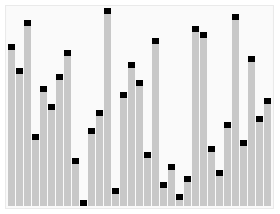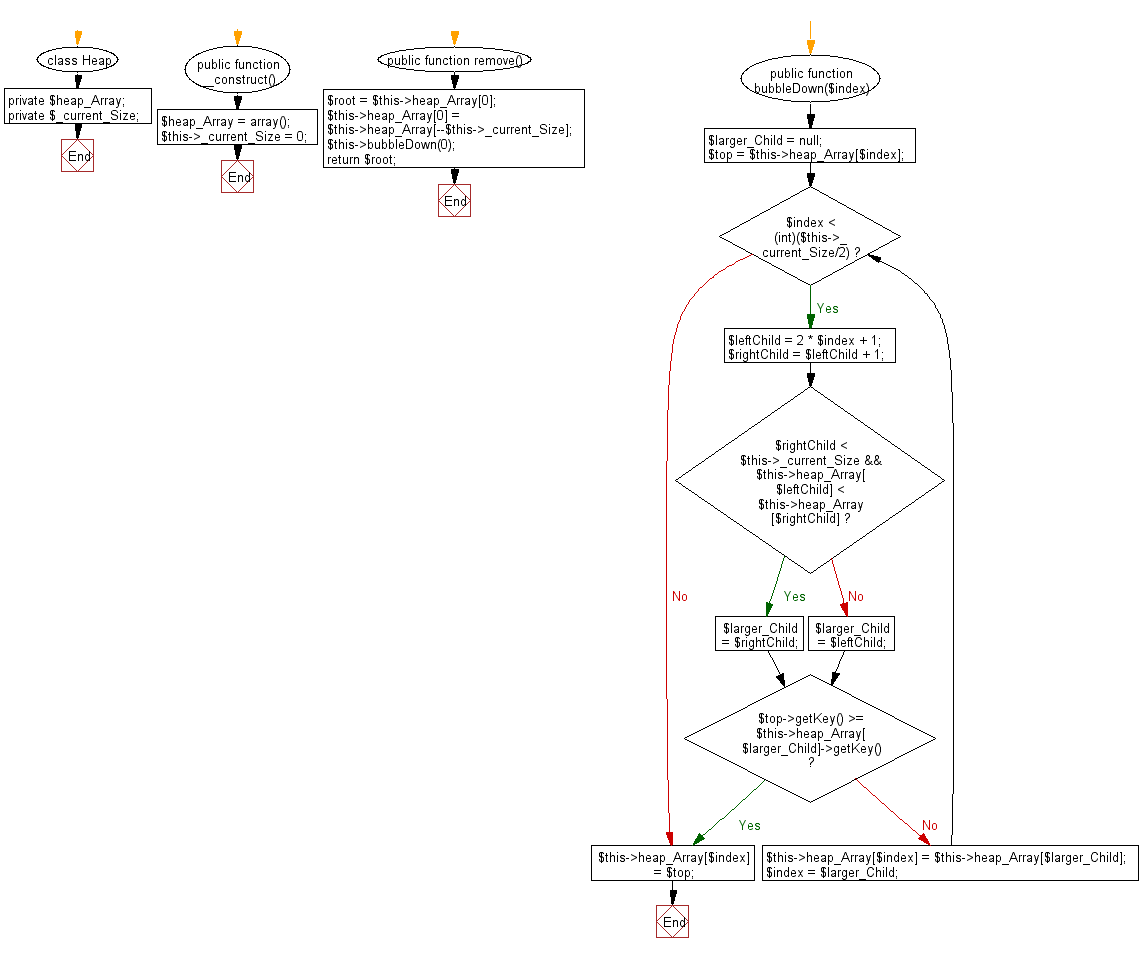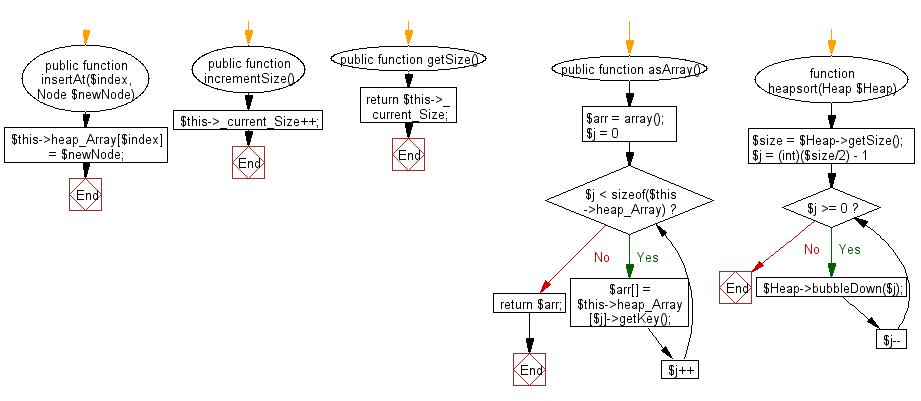﻿ PHP: Sort a list of elements using Heap sort - w3resource

# PHP Searching and Sorting Algorithm: Heap sort

## PHP Searching and Sorting Algorithm: Exercise-2 with Solution

Write a PHP program to sort a list of elements using Heap sort.

In computer science, heapsort (invented by J. W. J. Williams in 1964) is a comparison-based sorting algorithm. Heapsort can be thought of as an improved selection sort: like that algorithm, it divides its input into a sorted and an unsorted region, and it interactively shrinks the unsorted region by extracting the largest element and moving that to the sorted region. The improvement consists of the use of a heap data structure rather than a linear-time search to find the maximum. Although somewhat slower in practice on most machines than a well-implemented quicksort, it has the advantage of a more favorable worst-case O(n log n) runtime. Heapsort is an in-place algorithm, but it is not a stable sort.

A run of the heapsort algorithm sorting an array of randomly permuted values. In the first stage of the algorithm, the array elements are reordered to satisfy the heap property. Before the actual sorting takes place, the heap tree structure is shown briefly for illustration.Animation credits : RolandH

Sample Solution :

PHP Code :

``````<?php
class Node
{
private \$_i;

public function __construct(\$key)
{
\$this->_i = \$key;
}

public function getKey()
{
return \$this->_i;
}
}

class Heap
{
private \$heap_Array;
private \$_current_Size;

public function __construct()
{
\$heap_Array = array();
\$this->_current_Size = 0;
}

// Remove item with max key
public function remove()
{
\$root = \$this->heap_Array;
// put last element into root
\$this->heap_Array = \$this->heap_Array[--\$this->_current_Size];
\$this->bubbleDown(0);
return \$root;
}

// Shift process
public function bubbleDown(\$index)
{
\$larger_Child = null;
\$top = \$this->heap_Array[\$index]; // save root
while (\$index < (int)(\$this->_current_Size/2)) { // not on bottom row
\$leftChild = 2 * \$index + 1;
\$rightChild = \$leftChild + 1;

// find larger child
if (\$rightChild < \$this->_current_Size
&& \$this->heap_Array[\$leftChild] < \$this->heap_Array[\$rightChild]) // right child exists?
{
\$larger_Child = \$rightChild;
} else {
\$larger_Child = \$leftChild;
}

if (\$top->getKey() >= \$this->heap_Array[\$larger_Child]->getKey()) {
break;
}

// shift child up
\$this->heap_Array[\$index] = \$this->heap_Array[\$larger_Child];
\$index = \$larger_Child; // go down
}

\$this->heap_Array[\$index] = \$top; // root to index
}

public function insertAt(\$index, Node \$newNode)
{
\$this->heap_Array[\$index] = \$newNode;
}

public function incrementSize()
{
\$this->_current_Size++;
}

public function getSize()
{
return \$this->_current_Size;
}

public function asArray()
{
\$arr = array();
for (\$j = 0; \$j < sizeof(\$this->heap_Array); \$j++) {
\$arr[] = \$this->heap_Array[\$j]->getKey();
}

return \$arr;
}
}

function heapsort(Heap \$Heap)
{
\$size = \$Heap->getSize();
// "sift" all nodes, except lowest level as it has no children
for (\$j = (int)(\$size/2) - 1; \$j >= 0; \$j--)
{
\$Heap->bubbleDown(\$j);
}
// sort the heap
for (\$j = \$size-1; \$j >= 0; \$j--) {
\$BiggestNode = \$Heap->remove();
// use same nodes array for sorted elements
\$Heap->insertAt(\$j, \$BiggestNode);
}

return \$Heap->asArray();
}

\$arr = array(3, 0, 2, 5, -1, 4, 1);
echo "Original Array : ";
echo implode(', ',\$arr );
\$Heap = new Heap();
foreach (\$arr as \$key => \$val) {
\$Node = new Node(\$val);
\$Heap->insertAt(\$key, \$Node);
\$Heap->incrementSize();
}
\$result = heapsort(\$Heap);
echo "\nSorted Array : ";
echo implode(', ',\$result)."\n";
?>
```
```

Sample Output:

```Original Array : 3, 0, 2, 5, -1, 4, 1
Sorted Array : -1, 0, 1, 2, 3, 4, 5
```

Flowchart :PHP Code Editor:

Have another way to solve this solution? Contribute your code (and comments) through Disqus.

What is the difficulty level of this exercise?

Test your Programming skills with w3resource's quiz.

﻿